# Test Data AC3-12

## Description of Test Case Experiments

For the detailed study of particle-laden, swirling two-phase flows, a vertical test section with downward flow was chosen (Figure 2). In order to allow good optical access, a simple pipe expansion was selected as test section. Such a configuration has the advantage that the inlet conditions can be measured easily, which is important for performing numerical calculations. The complete test rig consists of two flow circuits (Figure 1) for the primary (6) and secondary annular flows (5), respectively. A blower (1) with a variable flow rate supplies these two pipe systems via a T-junction and a throttle valve (2) is used to adjust the flow rate at the primary inlet. The mass flow rates through the primary and annular inlets were obtained from two orifice flow meters (3). The secondary flow circuit is split into four smaller pipes which are connected radially to the swirl generator. The upper part of the swirl generator is constructed as a settling chamber, and the air passes over a number of screens and then moves radially inward across the radial swirl vanes. The swirl intensity of the annular flow may be adjusted continuously by turning the swirl vanes in the radial swirl generator (8). The primary flow circuit is connected to a pipe passing straight through the centre of the swirl generator. The dust particles are injected into the primary flow above the swirl generator by a particle feeder (4) with a variable-speed motor. Above the particle feeder, a reservoir (7) for the dust particles is installed.

The inlet configuration and the dimensions of the test section are shown in Figure 1. The test section consists of a 1.5 m long Plexiglas tube with an inner diameter of 194 mm. The end of the test section is connected to a stagnation chamber (11). As a result, an annular type of central recirculation bubble was established in the upper part of the test section.

The stagnation chamber is connected to a cyclone separator (13) and an additional paper filter to separate both the large dispersed phase particles and the seeding particles, which are used as tracers for measuring the gas velocity. These tracer particles are injected into both pipe systems before the swirl generator. To guarantee that the flow rate in the test section is independent of the pressure loss in the filter system, which may vary during the measurements, an additional blower (12) is used in connection with a bypass valve at the stagnation chamber (11).

## Measurement Technique

Particle size and velocity measurements were performed at several cross-sections within the test section, including the inlet using a one-component PDA (phase-Doppler anemometer). Details on the configuration of the applied PDA-system can be found in Sommerfeld and Qiu (1991 and 1993). In order to allow measurements of all three velocity components (axial velocity u, radial velocity v, and tangential velocity  w), the PDA system could be rotated and was mounted on a stepper-motor-controlled three-dimensional traversing system (Figure 1). The receiving optics was always mounted at an angle of 30° from the forward scattering direction. To avoid strong laser-beam deflections and a realignment of the receiving optics for every measuring point, the Plexiglas test section had several slits which were covered from the inside by 100 µm thick glass plates. This results in negligible beam distortion, especially when the radial and axial velocities are measured, where the receiving optics have to be mounted in such a way that the optical axis of the receiving optics is oblique to the walls of the test section. For each velocity component, different test sections with different slit locations were used, which allowed the appropriate installation of the PDA receiving optics and the measurement of particle size-velocity correlation for each velocity component.

In order to allow simultaneous measurements of gas and particle velocities, the flow was additionally seeded with small, spherical tracer particles (Ballotini, type 7000). This seeding was injected into both the primary and the annular jets far upstream the inlet to the test section. Since the size distribution of the particles ranges up to about 10 µm, a phase discrimination procedure was employed which ensured that only tracer particles up to a maximum of 4 µm are sampled for determining the gas velocity. This procedure resulted in a measured mean diameter of about 1.5 µm for the validated signals from the tracer particles. A detailed description of the phase discrimination procedure was given previously by Sommerfeld and Qiu (1991).

The particle mass flux was measured separately with the single-detector receiving system. The receiving optics was positioned 90° off-axis from the forward scattering direction in order to obtain an exact demarcation of the measuring volume. At each measuring point, the number of particles N traversing the control volume was counted within a certain time period Δt, and the particle velocity was measured simultaneously. For these measurements the transient recorder was also operated in the sequential mode, which ensured a real-time data acquisition, at least for the particle concentrations considered. During the storage of the 400 events, an internal clock was used to determine the effective measuring time. The total particle mass flux is then obtained with the cross-section of the control volume Ac, which was calculated from the optical configuration:

${f_{p}={\frac {N{\overline {m}}_{p}}{\Delta tA_{c}}}}$where ${\left.N\right.}$, ${\left.\Delta t\right.}$and ${{\overline {m}}_{p}}$are the counted number of particles, the total measuring time and the mean particle mass, respectively. The mean particle mass at a certain measuring location was calculated from the volume mean particle diameter obtained from the PDA size measurements. Due to the uncertainties in the determination of the cross-section of the control volume, the measured mass flux was corrected using the global mass balance. The total measured particle mass flow rate was obtained by integrating the mass flux profile at the inlet. In comparison with the global mass flow rate obtained by weighting the particles collected during a certain time period in the cyclone separator, a correction factor was determined and applied to the mass flux measurements at all other cross-sections. The integration of the measured and corrected mass flux profiles revealed that the error in the particle mass flow rate was in the range ( 20% for the various profiles. This rather large error was caused mainly by the poor resolution of the measurements in the near-wall region, where the integration area is the largest in the circular cross-section of the pipe. Furthermore, the particle mass flux was separated to give the positive and negative fluxes, which gives additional information about the mass and number of particles having negative velocities. For this separation, the particle volume mean diameter for particles with only positive and negative axial velocity was determined from the PDA measurements.

Measurements of the three velocity components were conducted at 8 cross-sections downstream of the inlet (i.e. 3, 25, 52, 85, 112, 155, 195 and 315 mm). At each measuring location, 2000 samples were taken to obtain the gas velocity and the associate rms values. In order to achieve reasonably accurate velocity statistics for the particle phase in the different size classes, 18,000 samples were acquired. The total measuring time for each location was between 15 – 30 min, which was strongly dependent on the local particle concentration. The maximum measurable particle size range, between 0 – 123.8 µm, was resolved by 40 classes, each 3.1 µm in width. Besides the information on the change in the particle size distribution throughout the flow field, the stored data of particle size and velocity could be reprocessed after the measurement in order to give the particle mean velocity in certain size classes.

## Measurement Errors

Since the three velocity components were measured with a single component PDA-system the measurement errors are very small; i.e. less than about 5%. For the measurement of the gas-phase velocity small spherical tracer particles were added. For identifying accurately the tracer signals a maximum phase angle was set whereby it was guaranteed that the tracer particles were smaller than about 3 µm (Sommerfeld and Qiu 1991). Such particles are able to follow the fluctuations of the gas flow. To determine the gas phase mean and rms velocities 2000 signals were collected.

Errors in particle sizing may occur due to fluctuations in the phase size relation, especially for particles smaller than about 50 µm (Sommerfeld and Tropea 1999). Additional errors may be caused by the so-called trajectory ambiguity. These errors are however difficult to specify.

Since for the particle phase 20,000 signal pairs were collected at each measurement location, the obtained size distributions were reasonable smooth. Therefore, the derived mean particle diameter should have an error of around ±5%. The measured size-velocity correlations are also very smooth for the major part of the size distribution around the modal value. Only near the edges of the size distribution with a lower number of samples some fluctuations are observed (Sommerfeld and Qiu 1991).

The procedure for measuring the particle mass flux and the associated errors are discussed in the previous section.

## Flow and Inlet Conditions

In the present experiments, two flow conditions with different flow rates at the particle-laden primary inlet were considered. The resulting maximum gas velocities in the primary jet for the two cases were 12.5 and 7.4 m/s, respectively. The flow rate in the annular inlet was adjusted to give a maximum velocity of about 18 m/s. The maximum tangential velocities for both cases were about 13 m/s, corresponding to a swirl vane angle of 30°. The resulting swirl number was about 0.5 in both cases.

The associated mass flow rates for the gas and the particles, the flow Reynolds number, the swirl number and other experimental conditions are listed in Table 1. The mass flow rates of the primary and secondary annular jets were calculated from the pressure drops across the orifice flow meters. The flow Reynolds number was obtained with the total volume flow rate at the inlet and the outer diameter of the annulus (D3 = 64 mm). The swirl number was calculated as the ratio of the axial flux of angular momentum to the axial flux of linear momentum, which was obtained by integration across both the primary and annular inlets.

Furthermore, the particle mass flow rates and the properties of the glass beads are given in Table 1. The particles have a smooth surface and are spherical in shape. Only less than about 2% of particles were non-spherical or fragments, which resulted in small errors in sizing the beads by the PDA. Such a particle material is ideal for PDA studies in particulate two-phase-flows. The particle size distribution obtained by a PDA measurement (18,000 samples) is given in Figure 3. Since during the experiment some of the smaller particles were not collected in the cyclone separator but were collected in the paper filter, the particle material was frequently renewed in order to guarantee that the particles always have the same size distribution. This was ensured by measuring the particle size distribution at the inlet from time to time. The effects of particle damage could not be observed in the present measurements.

 Case 1 Case 2 Table 1. Flow conditions and particle properties Air flow Gas temperature [K] 300 300 Gas density [kg/m3] 1.18 1.18 Dynamic viscosity [kg/(m s)] 18.4×10-6 18.4×10-6 Mass flow rate of the primary jet [g/s] 9.9 6.0 Mass flow rate of the secondary jet [g/s] 38.3 44.6 Ratio of mass flow rates secondary/primary 3.87 7.43 Inlet Reynolds number (with D3 = 64 mm 52400 54500 Swirl number 0.47 0.49 particle phase Particle mass flow rate [g/s] 0.34 1.0 Particle loading in the primary jet 0.034 0.17 particle properties Particle number mean diameter [µm] 45 Particle material density [kg/m3] 2500 Stokesian particle response time [ms] 15.2 Refractive index 1.52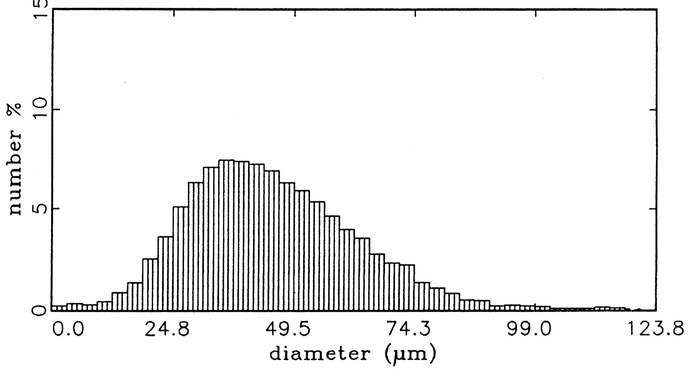Figure 3: Measured number-based size distribution of the spherical glass beads at the inlet to the test section (number mean diameter 45 μm).

## Measurement Data

Experiments were conducted for two swirl flow cases (Table 1). For each of the cases the inlet data (at z = 3 mm) and the profiles at cross-sections 25, 52, 85, 112, 155, 195 and 315 mm downstream of the inlet are collected by traversing the PDA in steps between 5 and 10 mm, except for the inlet which was scanned at a higher resolution (between 1 and 3 mm). All the data for the two cases are available at webpage of the institute MVT: http://www-mvt.iw.uni-halle.de/testfaelle/drallstroemung/.

These data sets (SWCASE1 and SWCASE2) include profiles for the gas phase velocities (all three velocity components) and profiles of particle phase properties averaged over all particle size classes (three velocity components, stream-wise particle mass flux and particle number mean diameter as well as its rms values). Moreover, profiles of the mean velocities of three particle size classes, namely 30, 45 and 60 μm, are provided.

## Overview of Experimental Results

The swirling flow structures established in the experiments exhibit closed central recirculation bubbles just downstream of the inlet and a rather short recirculation at the edge of the pipe expansion. Due to the strong spreading of the swirling jet, the reattachment length is considerably shorter than that for a similar pipe expansion flow without swirl.

An overview of the gas flow field and the particle behaviour in the two swirl flow conditions considered (cases 1 and 2) is given in Figure 4, where the gas-phase zero axial velocity lines, the dividing streamlines and the zero axial velocity lines for the particles (averaged over all particle classes) are shown. The width of the central recirculation bubble is almost identical for both cases, whereas the length is larger for case 2. As a result of the lower axial momentum of the primary jet in case 2, the recirculation bubble moves closer to the inlet. The slightly higher swirl number in this case also gives rise to a shorter reattachment length of the recirculation region at the edge of the pipe expansion, as compared with case 1.

The area of negative axial particle velocity exhibits an annular shape ranging from about 50 to 200 mm downstream of the inlet for swirl case 1. In case 2, where the particle initial momentum is lower, the particles have longer residence times in the flow and, therefore, respond faster to the flow reversal in the central recirculation bubble. The resulting area of negative particle axial velocities becomes wider and extends from 50 to about 250 mm in the stream-wise direction.

The measurements of the cross-sectional profiles for the mean gas and particle velocities, the velocity fluctuations and the development of the particle mass flux and the particle number mean diameter reveal the particle response and dispersion characteristics. The measurements of the particle mass flux for the two flow situations already show considerable differences (Figure 5). The maximum of the mass flux near the centreline remains up to 200 mm downstream of the inlet for case 1. While in case 2, the particles begin to accumulate near the wall quite early (from z = 112 mm), and the maximum of the mass flux appears near the centreline only up to about z = 112 mm. Furthermore, it may be seen that the recirculating particle mass (i.e. negative mass flux) is considerably higher for swirl case 2. In addition, the fraction of recirculating particles is found to be higher in the initial part of the recirculation bubble for this case. This indicates that this flow condition is more suitable for pulverized coal combustion when the diameter of the coal particles is rather large and where the devolatilization occurs in the central recirculation bubble. This effect may considerably reduce NOx formation.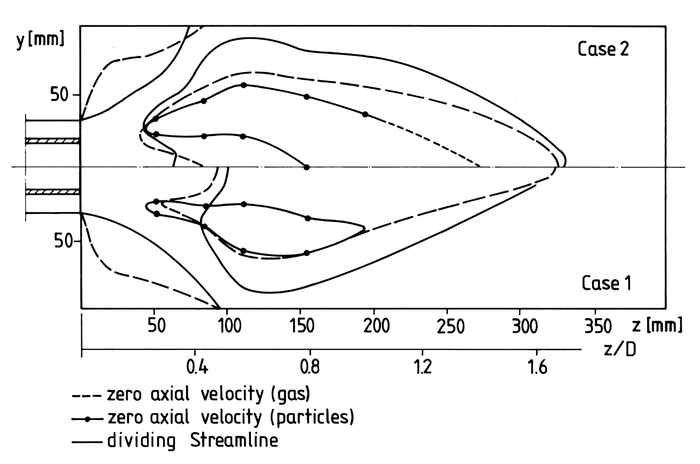Figure 4: General flow characteristics for the two swirling cases showing the zero axial velocity line for gas and particle phase as well as the dividing stream lines.

Due to the different responses of the differently sized particles to the flow reversal, the flow turbulence and to the centrifugal effects, a considerable separation of the particle phase is observed in the two flow conditions considered (see Sommerfeld and Qiu 1993). These effects result in a remarkable change in the particle size distribution and, hence, in the particle number mean diameter throughout the flow field. Due to the higher inertia of the larger particles, they remain concentrated in the core region while the smaller ones are entrained into the co-flowing annulus. This results in a continuously increasing number mean particle diameter in the stream-wise direction in the core region of the flow for both cases (Figure 6). As already demonstrated by numerically simulated particle trajectories (Sommerfeld and Qiu 1993), the particles have more time to respond to the flow reversal and the centrifugal forces in case 2, due to their lower initial velocity. Hence, a minimum in the particle mean diameter is already observed in the core region of the central recirculation region at z = 195 mm, which implies that the majority of larger particles have also been transported towards the wall. Since the particle mass flux is already very low at this location, only some smaller recirculating particles, or those which have been reflected from the wall, were recorded here.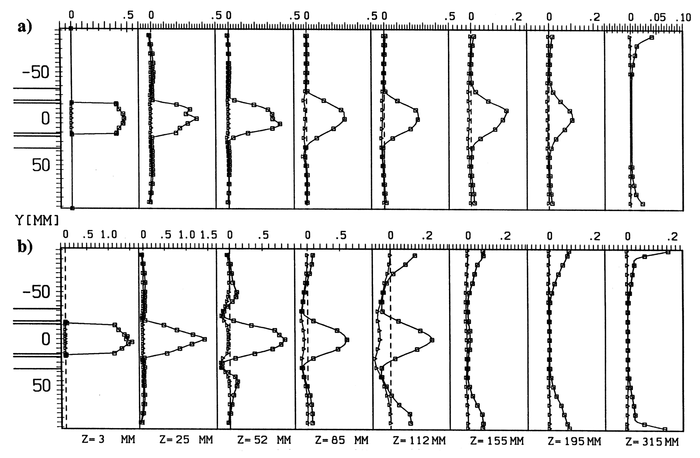Figure 5: Measured particle mass flux profiles [kg/m2s] (□, total axial mass flux; △, negative axial mass flux): (a) case 1; (b) case 2.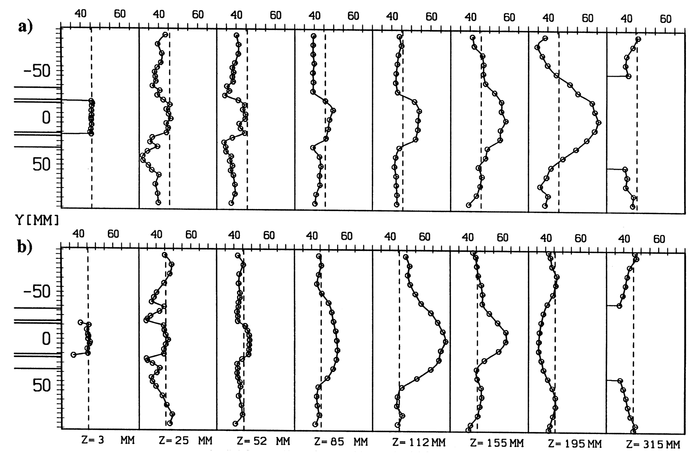Figure 6: Measured profiles of local particle number mean diameter [μm]: (a) case 1; (b) case 2.

In order to demonstrate the particle velocity characteristics in the two flow conditions considered, the measured radial profiles of the axial mean velocity and the corresponding velocity fluctuations for both cases are shown in Figures 7 and 8; i.e. for the gas phase and the three particle size classes (i.e. 30, 45 and 60 µm). These results again demonstrate the behaviour of particles with different diameters, which is strongly governed by inertial effects in this complex flow. The particles are not able to follow the rapid expansion of the air jets and the resulting deceleration of the flow. Hence, the particles maintain a higher velocity than the air flow in the core region of the test section and within the central recirculation bubble. The larger particles (i.e. 60 µm) have the highest velocities due to their larger inertia. In swirl case 2, it is observed that within the circulation bubble, the particles acquire higher negative axial velocities than in case 1.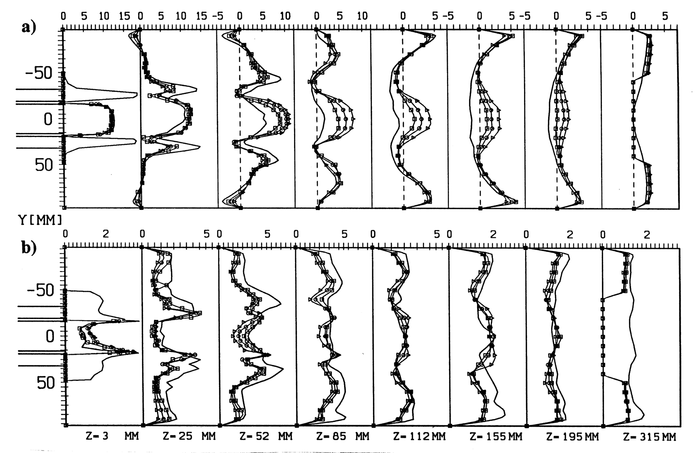Figure 7: Measured profiles of axial mean velocities (a) and rms-values (b) for air and particles in [m/s] for Case 1: — air, □ 30 μm particles, ◯ 45 μm particles, △ 60 μm particles.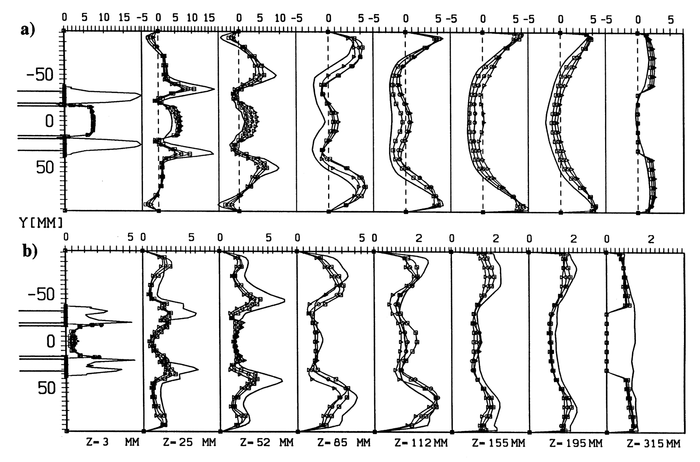Figure 8: Measured profiles of axial mean velocities (a) and rms-values (b) for air and particles in [m/s] for Case 2: — air, □ 30 μm particles, ◯ 45 μm particles, △ 60 μm particles.

An interesting phenomenon is observed for the velocity fluctuations of the particles in the stream-wise direction (Figures 7 and 8). For both cases one finds regions where the rms value is higher for the particles than for the gas phase. This, of course, cannot result from the response of the particles to the fluid turbulence. This effect is a consequence of the so-called "history effect" (Sommerfeld & Qiu 1991) and is caused by the fact that particles from completely different directions cross a certain location within the recirculation bubble and near its edge. This means that particles issuing straight from the inlet, which still have positive velocities, and recirculating particles, which come from further downstream, are sampled at these locations. Therefore, this effect results in broader velocity distributions for the particles compared to the gas velocity distribution. Especially in case 1, this effect is more pronounced for the larger particles. Furthermore, it is obvious that in certain regions the velocity fluctuations of the larger particles are higher than for the smaller ones (e.g. case 1 at z = 112 and 155 mm, case 2 at z = 52 and 85 mm), which is again a result of inertial effects.

The radial and tangential mean velocity profiles for the gas phase and the three classes of particles are shown in Figure 9 for case 2. The radial velocity profiles (Figure 9(a)) could only be measured for half a cross-section due to limited optical access. As a result of the rapid expansion of the jets, the gas and particles move radially outward up to approx. z = 85 mm. As a result of their inertia, the particles have slightly higher radial mean velocities than the gas phase. From z = 112 downstream of the inlet the particles move inward, since they have rebounded from the wall.

The tangential velocity profiles of the gas and particles (Figure 9(b)) demonstrate that the particles lag behind the rotation of the gas flow. From the beginning of the central recirculation bubble at typical vortex structure may be identified where, in the core region, a solid body rotation develops for the gas and particle phases.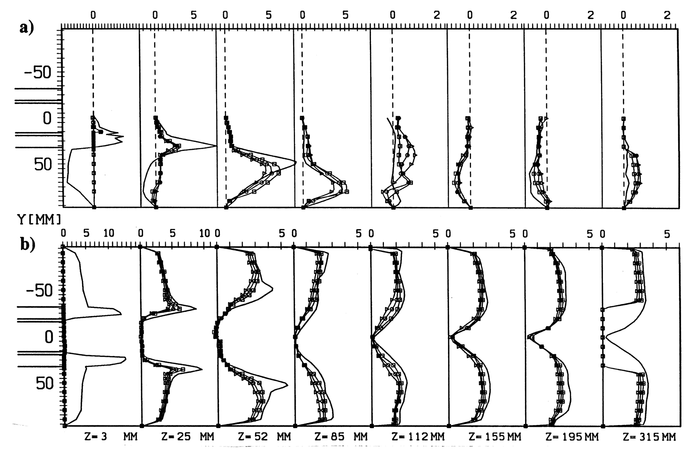Figure 9: Measured profiles of radial (a) and tangential (b) mean velocities for air and particles in [m/s] for Case 2: — air, □ 30 μm particles, ◯ 45 μm particles, △ 60 μm particles.

Contributed by: Martin Sommerfeld — Martin-Luther-Universität Halle-Wittenberg